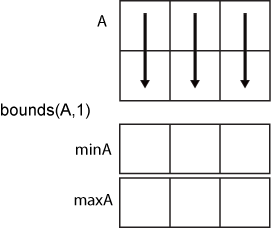bounds

Minimum and maximum values of an array

Description

example

[minA,maxA] = bounds(A) returns the minimum value minA and maximum value maxA in an array. minA is equivalent to min(A) and maxA is equivalent to max(A).

example

[minA,maxA] = bounds(A,'all') computes the minimum and maximum values over all elements of A. This syntax is valid for MATLAB® versions R2018b and later.

example

[minA,maxA] = bounds(A,dim) operates along the dimension dim of A. For example, if A is a matrix, then bounds(A,2) returns column vectors minA and maxA containing the minimum and maximum values in each row.

example

[minA,maxA] = bounds(A,vecdim) computes the minimum and maximum values based on the dimensions specified in the vector vecdim. For example, if A is a matrix, then bounds(A,[1 2]) returns the minimum and maximum values over all elements in A, since every element of a matrix is contained in the array slice defined by dimensions 1 and 2.

example

[minA,maxA] = bounds(___,nanflag) specifies whether to include or omit NaN values when determining the minimum and maximum values. bounds(A,'omitnan') ignores NaN values. If any element of A is NaN, then bounds(A,'includenan') returns NaN for both minA and maxA. The default behavior is 'omitnan'.

Examples

collapse all

Simultaneously compute the minimum and maximum values of a vector.

A = [2 4 -1 10 6 3 0 -16];
[minA,maxA] = bounds(A)
minA = -16
maxA = 10

Compute the minimum and maximum values in each row of a matrix.

A = magic(4)
A = 4×4

16     2     3    13
5    11    10     8
9     7     6    12
4    14    15     1

[minA,maxA] = bounds(A,2)
minA = 4×1

2
5
6
1

maxA = 4×1

16
11
12
15

Create a 3-D array and compute the minimum and maximum values in each page of data (rows and columns).

A(:,:,1) = [2 4; -2 1];
A(:,:,2) = [9 13; -5 7];
A(:,:,3) = [4 4; 8 -3];
[minA1,maxA1] = bounds(A,[1 2]);
minA1
minA1 =
minA1(:,:,1) =

-2

minA1(:,:,2) =

-5

minA1(:,:,3) =

-3

maxA1
maxA1 =
maxA1(:,:,1) =

4

maxA1(:,:,2) =

13

maxA1(:,:,3) =

8

Starting in R2018b, to compute the bounds over all dimensions of an array, you can either specify each dimension in the vector dimension argument, or use the 'all' option.

[minA2,maxA2] = bounds(A,[1 2 3])
minA2 = -5
maxA2 = 13
[minAall,maxAall] = bounds(A,'all')
minAall = -5
maxAall = 13

Include and ignore NaN elements of a vector when computing its minimum and maximum values.

Ignore NaN values when computing the minimum and maximum values of a vector, which is the default.

A = [2 NaN 6 -5 0 NaN 10];
[minA1,maxA1] = bounds(A)
minA1 = -5
maxA1 = 10

Use the 'includenan' option to include NaN values, which causes bounds to return NaN for both the minimum and maximum values of A.

[minA2,maxA2] = bounds(A,'includenan')
minA2 = NaN
maxA2 = NaN

Input Arguments

collapse all

Input array, specified as a vector, matrix, or multidimensional array.

Data Types: single | double | int8 | int16 | int32 | int64 | uint8 | uint16 | uint32 | uint64 | logical | categorical | datetime | duration
Complex Number Support: Yes

Dimension to operate along, specified as a positive integer scalar. If no value is specified, then the default is the first array dimension whose size does not equal 1.

Consider a matrix A.

• bounds(A,1) computes the minimum and maximum values in each column.• bounds(A,2) computes the minimum and maximum values in each row.Vector of dimensions, specified as a vector of positive integers. Each element represents a dimension of the input array. The lengths of the output in the specified operating dimensions are 1, while the others remain the same.

Consider a 2-by-3-by-3 input array, A. Then [minA,maxA] = bounds(A,[1 2]) returns a 1-by-1-by-3 array for both minA and maxA. The elements of minA and maxA are the minimum and maximum values in the corresponding page of A, respectively.NaN condition, specified as one of these values:

• 'omitnan' — Ignore all NaN values in the input. If the input contains only NaN values, then bounds returns NaN for both minA and maxA.

• 'includenan' — Include NaN values. If any element of the input is NaN, then bounds returns NaN for both minA and maxA.

Output Arguments

collapse all

Minimum value, specified as a vector, matrix, or multidimensional array.

Maximum value, specified as a vector, matrix, or multidimensional array.